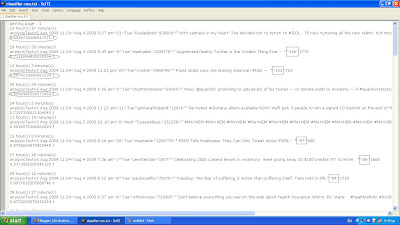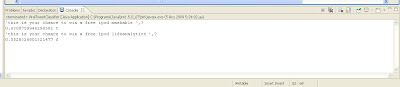# A computer program predicts Viral Tweets

In the previous post we have seen that the author of a Tweet is the most important factor for making a viral Tweet. This time we will use text mining to score Tweets and see how much viral they could become. Each Tweet is fed to a computer program (an algorithm) and the algorithm responds with the probability each Tweet has to become viral (we assume that when a Tweet receives more than 30 Re-Tweets it is considered viral).

The information that is given to the algorithm is the text of the Tweet and its author. Many other parameters can be taken into consideration such as the time that the Tweet has been posted, the type of the Tweet (e.g., politics, technology, health) or even whether this Tweet is part of a novel subject. Here is the output of the software that performs the predictions:The number of Re-Tweets is shown in squares. Pay also close attention to the circled text shown above. For each Tweet the most probable outcome is given (‘t’= Tweet will become viral, ‘f’=otherwise) and a confidence for each prediction is given as a number from 0 to 1. As an example, the first Tweet shown above was posted from Paula Abdul saying that she will not return to American Idol. The

In the previous post we have seen that the author of a Tweet is the most important factor for making a viral Tweet. This time we will use text mining to score Tweets and see how much viral they could become. Each Tweet is fed to a computer program (an algorithm) and the algorithm responds with the probability each Tweet has to become viral (we assume that when a Tweet receives more than 30 Re-Tweets it is considered viral).

The information that is given to the algorithm is the text of the Tweet and its author. Many other parameters can be taken into consideration such as the time that the Tweet has been posted, the type of the Tweet (e.g., politics, technology, health) or even whether this Tweet is part of a novel subject. Here is the output of the software that performs the predictions:The number of Re-Tweets is shown in squares. Pay also close attention to the circled text shown above. For each Tweet the most probable outcome is given (‘t’= Tweet will become viral, ‘f’=otherwise) and a confidence for each prediction is given as a number from 0 to 1. As an example, the first Tweet shown above was posted from Paula Abdul saying that she will not return to American Idol. The algorithm predicts with a confidence of 63.38% that what Paula Abdul posted will be interesting (and it actually was).

The predictive model has an overall accuracy of 72.88% in predicting which Tweets will be viral in a total of 59 Tweets. An example of an incorrect prediction can be seen at the 4th circle from the top. The algorithm gave a 53.66% confidence that this Tweet will not become viral but actually this was a viral Tweet.

You can find the text file of the actual run from the algorithm here.

By looking the text file, results metrics such as TP (True positives) versus FP (False positives) can be calculated. It is also interesting to see how the algorithm switches to negative predictions when the number of Re-Tweets of each Tweet become less than 30.

Even though the example given here is very simplistic – and optimistic – the application of a tool of this kind for PR, marketing and branding could prove very useful. Marketeers can try different messages and see what impact each message is likely to have. Consider the following run that shows that @mashable is more influential than @lifeanalytics :The following run shows that specific keywords raise our chances in making a Viral Tweet :